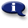# .

## Syllabus Information

Summer 2022
Oct 07,2022Use this page to maintain syllabus information, learning objectives, required materials, and technical requirements for the course.

Syllabus Information
CH 150 - Preparatory Chemistry
Associated Term: Summer 2022
Learning Objectives: Upon successful completion of this course, the student should be able to: 1. Use measurements written in scientific notation 2. Analyze uncertainty in measurements, apply rules of significant figures 3. Solve dimensional analysis (unit conversion) questions, apply problem solving techniques to a variety of real life questions 4. Calculate density or use density as a conversion factor 5. Distinguish between different types of compounds 6. Name chemicals given a formula or write a formula given a name 7. Identify the components of an atom, identify isotopes 8. Identify when the concept of a mole is used correctly or incorrectly and use a mole to solve a variety of chemistry questions 9. Calculate molar mass, % composition, empirical and molecular formulas 10. Write and balance chemical equations, distinguish between different types of reactions 11. Use and apply previous concepts to solve stoichiometry problems 12. Observe the properties of gases, solve gas law questions 13. Determine the concentration of a solution, apply stoichiometry concepts to solutions 14. Be prepared to take and succeed in CH 221
Required Materials:
Technical Requirements: